#Function Repository Resource:

# BinarySerializeWithDefinitions

Serialize an expression along with any dependent definitions

Contributed by: Richard Hennigan (Wolfram Research)
 ResourceFunction["BinarySerializeWithDefinitions"][expr] serializes expr to a ByteArray along with its dependent definitions.

## Details and Options

ResourceFunction["BinarySerializeWithDefinitions"] has the following options:
 Method Automatic details of serialization methods to use PerformanceGoal Automatic aspects of performance to try to optimize
Possible settings for PerformanceGoal include:
 "Speed" optimize for serialization and deserialization speed "Size" optimize for smallness of serialized output Automatic automatically pick serialization strategy
Possible settings for Method include:
 { typespec 1 → enc 1 , … } specify encodings for particular types Automatic pick encodings automatically based on data
Possible forms for the typespeci include:
 "PackedArrayIntegerType" integer packed arrays "PackedArrayRealType" real-valued packed arrays "PackedArrayComplexType" complex-valued packed arrays
Possible settings for "PackedArrayIntegerType" include "Integer8", "Integer16", "Integer32" and "Integer64" (64-bit systems only).
Possible settings for "PackedArrayRealType" include "Real32" and "Real64".
Possible settings for "PackedArrayComplexType" include "Complex64" and "Complex128".
The default for the enci encoding specifications is Automatic, in which case ResourceFunction["BinarySerializeWithDefinitions"] will use a type that fits the data it is given.
ResourceFunction["BinarySerializeWithDefinitions"] uses the WXF format.

## Examples

### Basic Examples

Serialize an expression with dependent definitions:

 In:=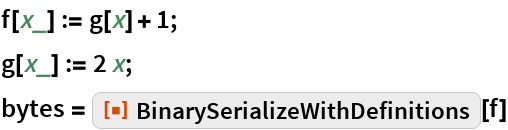Out=Definitions are restored when deserialized:

 In:=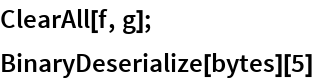Out=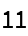### Generalizations and Extensions

The serialized expression is an evaluation that restores definitions before returning the output:

 In:=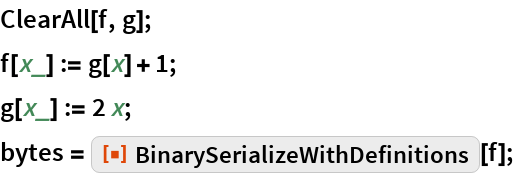In:=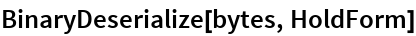Out=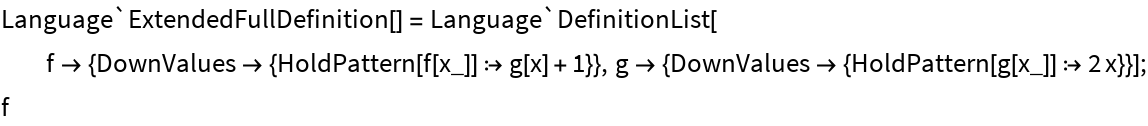BinarySerialize by itself does not preserve definitions:

 In:=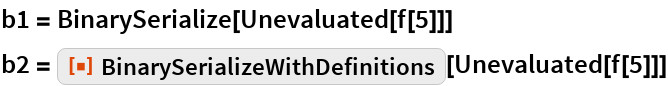Out=Out=In:=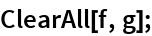In:=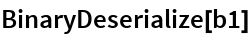Out=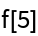In:=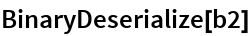Out=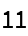### Options

#### PerformanceGoal

Serialize a Dataset:

 In:=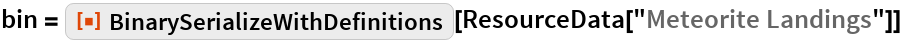Out=Serialize the same Dataset with PerformanceGoal set to "Size":

 In:=Out=Both forms represent the same expression:

 In:=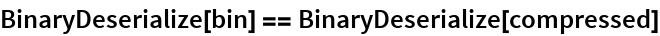Out=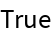#### Method

Create a packed array of integer values:

 In:=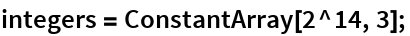By default, BinarySerializeWithDefinitions uses the smallest integer type that fits the data:

 In:=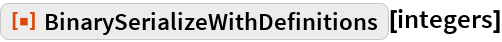Out=Serialize the packed array using a bigger integer type:

 In:=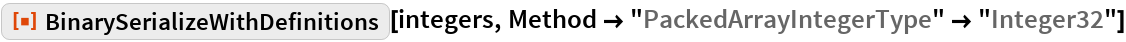Out=Create a packed array of real values:

 In:=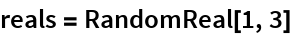Out=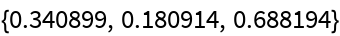Serialize the array:

 In:=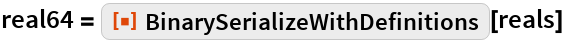Out=Serialize the array using machine floats, trading precision for a smaller output:

 In:=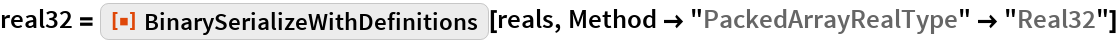Out=Create a packed array of complex values:

 In:=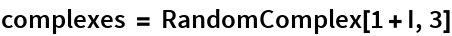Out=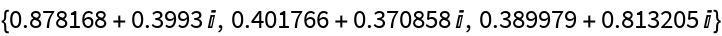Serialize the array:

 In:=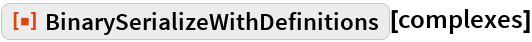Out=Serialize the array using lower precision:

 In:=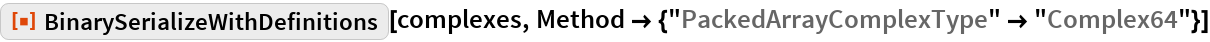Out=### Applications

CloudPut an evaluation that runs each time you use CloudGet on it:

 In:=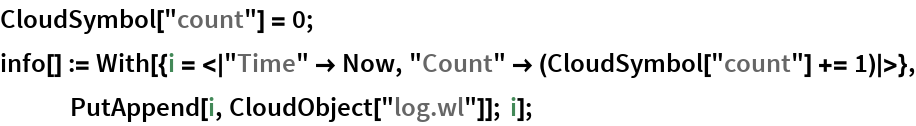In:=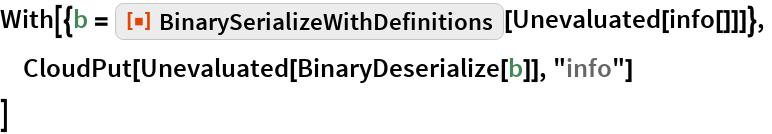Out=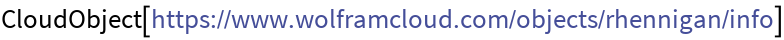In:=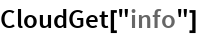Out=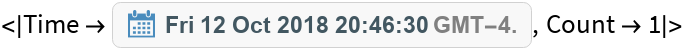In:=Out=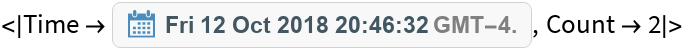Check the log:

 In:=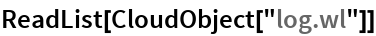Out=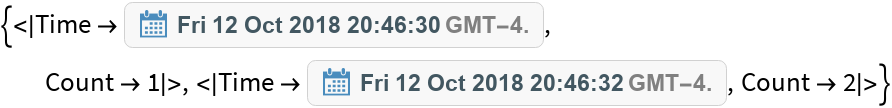### Properties and Relations

When there are no dependent symbols, BinarySerializeWithDefinitions will produce the same ByteArray as BinarySerialize:

 In:=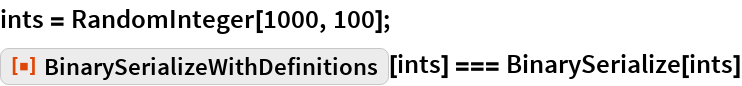Out=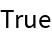The results are different when definitions need to be included:

 In:=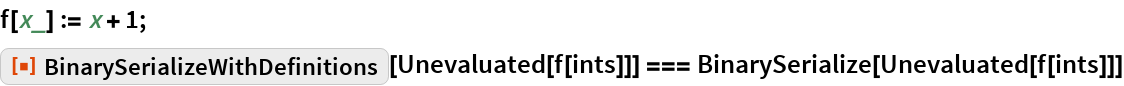Out=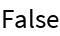### Possible Issues

For small expressions, definitions add a significant amount of overhead relative to the total size:

 In:=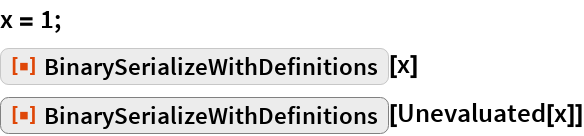Out=Out=This overhead becomes insignificant for larger expressions:

 In:=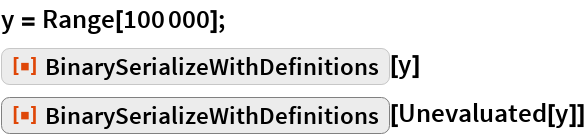Out=Out=## Requirements

Wolfram Language 11.3 (March 2018) or above